International
Tables for
Crystallography
Volume F
Crystallography of biological macromolecules
Edited by E. Arnold, D. M. Himmel and M. G. Rossmann

International Tables for Crystallography (2012). Vol. F, ch. 2.2, p. 69

## Section 2.2.5. Quality indicators for phase determination

H. M. Einspahra* and M. S. Weissb

aPO Box 6483, Lawrenceville, NJ 08648–0483, United States, and bHelmholtz-Zentrum Berlin für Materialien und Energie, Macromolecular Crystallography (HZB-MX), Albert-Einstein-Str. 15, D-12489 Berlin, Germany
Correspondence e-mail:  hmeinspahr@yahoo.com

### 2.2.5. Quality indicators for phase determination

| top | pdf |

Once the isomorphous or anomalous substructure has been successfully determined, it can be used as reference point for the calculation of phases. The quality of the resulting phases is dependent on the strength of the isomorphous or anomalous signal and the completeness and correctness of the isomorphous or anomalous substructure.

Cullis R factor, RCullis. The Cullis R factor (Cullis et al., 1961) for phase determination by isomorphous replacement is defined as the ratio between the lack-of-closure errorP) [equation (2.2.5.1a)below] and the isomorphous difference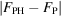. It is the most useful signal for a usable heavy-atom derivative. Values < 0.6 for centrosymmetric data are excellent, while values < 0.9 are still usable.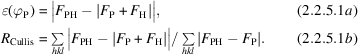Anomalous Cullis R factor, RCullis,ano. The Cullis R factor for phase determination by anomalous dispersion is defined as the ratio between the lack-of-closure error and the observed anomalous difference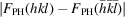. The lack-of-closure error in the anomalous-dispersion case is the difference between the observed anomalous difference and the calculated anomalous difference 2FH sin αP, where αP is the protein phase. A value of RCullis,ano < 1.0 suggests that a contribution to the phasing from the anomalous data is likely (MLPHARE program documentation; Collaborative Computational Project, No. 4, 1994).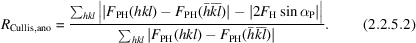Phasing power, PPiso. The isomorphous phasing power PPiso for phase determination by isomorphous replacement is defined for a particular pair of native and heavy-atom-derivative data sets as the ratio of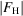andP), whereis the calculated amplitude of the heavy-atom structure factor andP) is the lack-of-closure error [equation (2.2.5.1a)]. FP + FH is a vector sum of the calculated structure factor FH and the structure factor FP.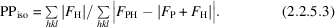There is another, slightly different, definition of PPiso, which is implemented in the program SOLVE. Here, PPiso is defined as the ratio of the r.m.s. of thevalues and the r.m.s. of the lack-of-closure errorsP). For each reflection hkl, a weighted average ofP) is calculated by integratingP) over the whole phase circle.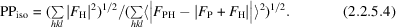Note. Owing to the cancelling out of the factor N1/2 in the numerator and denominator, the latter PPiso formula does not appear as a ratio of r.m.s. values at first sight.

Anomalous phasing power, PPano. The anomalous phasing power PPano for phase determination by anomalous-dispersion methods is defined as the ratio of the sum of calculated anomalous differences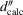and the sum of estimated standard uncertainties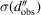in the measurement of these anomalous differences: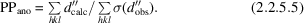As with PPiso (see above), the program SOLVE uses a slightly different definition of PPano. Here, the anomalous phasing power is defined as the ratio of the r.m.s. of thevalues and the r.m.s. of. For this, a weighted average ofis computed by integrating over the whole phase circle for each reflection.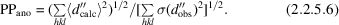Note. As above in the PPiso formula, the factors N1/2 in the numerator and denominator cancel out.

Figure of merit (f.o.m.), m. The figure of merit m is a term used in a number of contexts in X-ray crystallography. In its most common use, it is defined as the weight applied to an individual structure-factor amplitude that, in conjunction with its best phase, gives rise, in a Fourier synthesis, to the electron-density map with the minimum level of noise (Blow & Crick, 1959). Typically, m is given as an average value over all reflections in the data set or in a given resolution shell.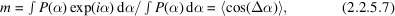where P(α) is the probability of the phase α, initial or refined, being the best phase and Δα = αbest − α is the error in the phase angle at α. The integration is from 0 to 2π and values for m range from 0 to 1.

### References

Blow, D. M. & Crick, F. H. C. (1959). The treatment of errors in the isomorphous replacement method. Acta Cryst. 12, 794–802.
Collaborative Computational Project, Number 4 (1994). The CCP4 suite: programs for protein crystallography. Acta Cryst. D50, 760–763.
Cullis, A. F., Muirhead, H., Perutz, M. F., Rossmann, M. G. & North, A. C. T. (1961). The structure of haemoglobin. VIII. A three-dimensional Fourier synthesis at 5.5 Å resolution: determination of the phase angles. Proc. R. Soc. London Ser. A, 265, 15–38.